# What, Why and Which?? Activation Functions

While studying neural network, we often come across the term — “Activation functions”. What are these activation functions?

### 1. The basic idea how a neural network works —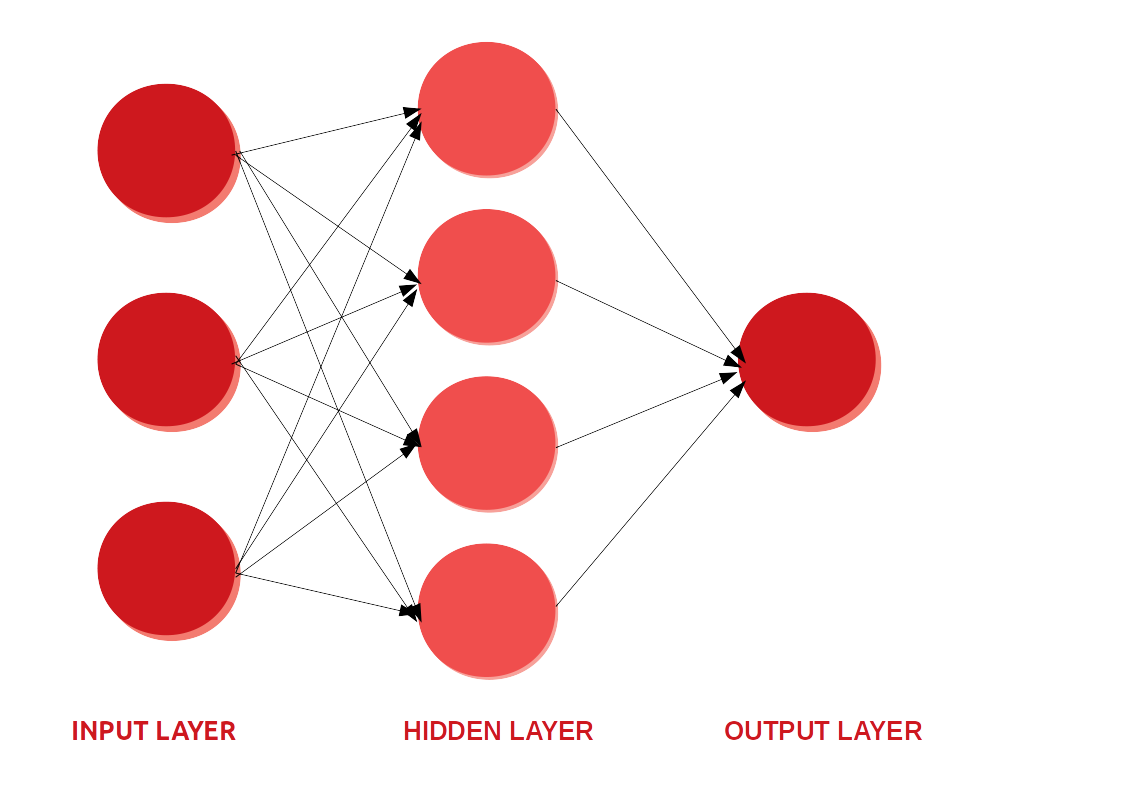Simple neural network

Neural networks are information processing paradigms inspired by the way biological neural systems process data. It contains layers of interconnected nodes or neurons arranged in interconnected layers. The information moves from the input layer to the hidden layers. In a simple case of each layer, we just multiply the inputs by the weights, add a bias and apply an activation function to the result and pass the output to the next layer. We keep repeating this process until we reach the last layer. Hidden layers fine-tune the input weightings until the neural network’s margin of error is minimal. We would update the weights and biases of the neurons on the basis of the error.

### 2. What is an Activation function?

The activation function is a mathematical “gate” in between the input feeding the current neuron and its output going to the next layer. They basically decide whether the neuron should be activated or not.

Consider a neuron —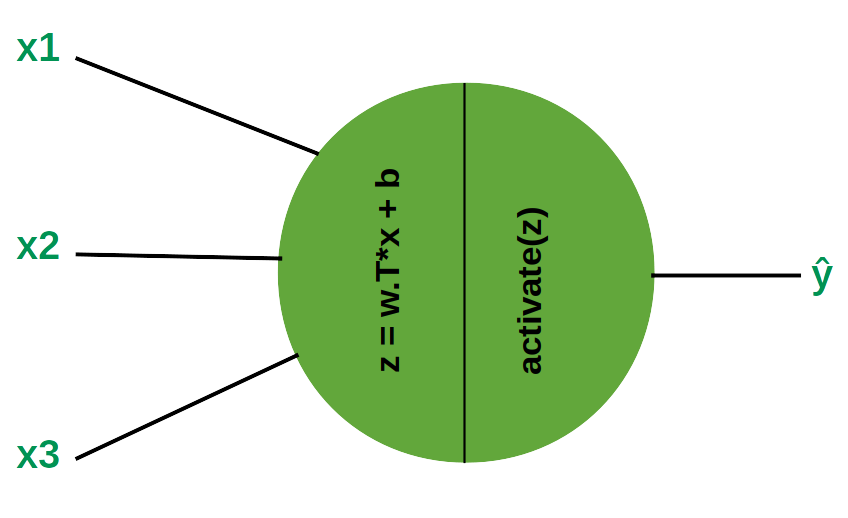A Neuron

### 3. Why do we use an activation function ?

If we do not have the activation function the weights and bias would simply do a linear transformation. A linear equation is simple to solve but is limited in its capacity to solve complex problems and have less power to learn complex functional mappings from data. A neural network without an activation function is just a linear regression model.

Generally, neural networks use non-linear activation functions, which can help the network learn complex data, compute and learn almost any function representing a question, and provide accurate predictions.

#### 3.1 Why use a non-linear activation function?

If we were to use a linear activation function or identity activation functions then the neural network will just output a linear function of input. And so, no matter how many layers our neural network has, it will still behave just like a single layer network because summing these layers will give us another linear function which is not strong enough to model data.

Also our activation function should be differentiable.

### 4. Types of Activation Function

The Activation Functions can be basically divided into 2 types-

1. Linear or Identity Activation Function
2. Non-linear Activation Functions

#### 4.1 Linear or Identity Activation Function

It takes the inputs, multiplied by the weights for each neuron, and creates an output signal proportional to the input.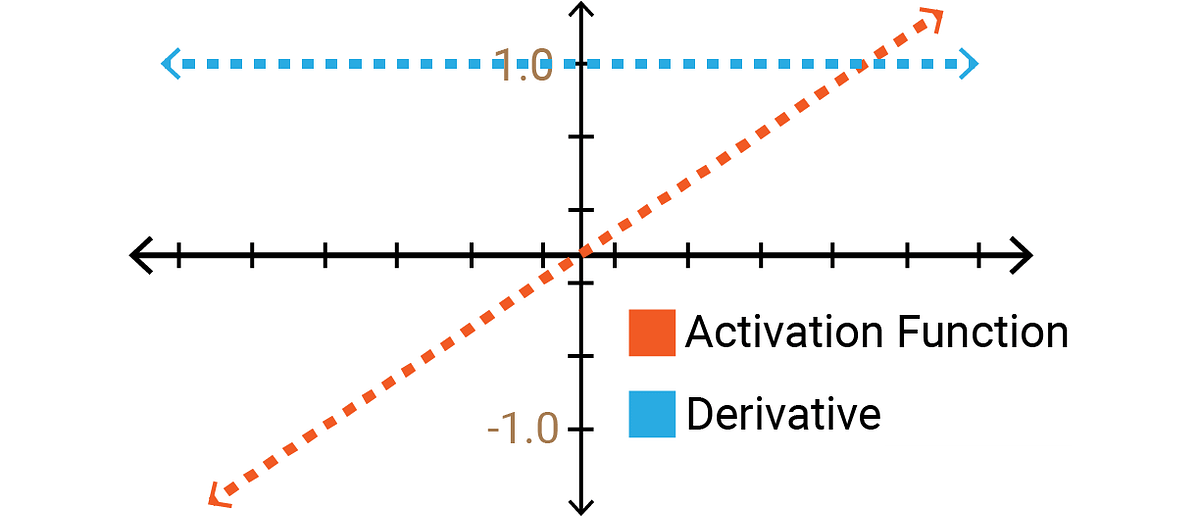Identity function and it’s derivative

Equation: f(x) = x

Derivative: f’(x) = 1

Range: (-∞, +∞)

Two major problems:

1. Back-propagation is not possible — The derivative of the function is a constant, and has no relation to the input, X. So it’s not possible to go back and understand which weights in the input neurons can provide a better prediction.
2. All layers of the neural network collapse into one — with linear activation functions, no matter how many layers in the neural network, the last layer will be a linear function of the first layer

#### 4.2 Non-linear Activation Function

Modern neural network models use non-linear activation functions. They allow the model to create complex mappings between the network’s inputs and outputs, which are essential for learning and modeling complex data, such as images, video, audio, and data sets which are non-linear or have high dimensionality.

Almost any process imaginable can be represented as a functional computation in a neural network, provided that the activation function is non-linear.

Non-linear functions address the problems of a linear activation function:

1. They allow back-propagation because they have a derivative function which is related to the inputs.
2. They allow “stacking” of multiple layers of neurons to create a deep neural network. Multiple hidden layers of neurons are needed to learn complex data sets with high levels of accuracy.

### 5. Some commonly used non-linear activation functions are —

#### 1. Sigmoid / Logistic

Sigmoid function gives an ‘S’ shaped curve. In order to map predicted values to probabilities, we use the sigmoid function. The function maps any real value into another value between 0 and 1.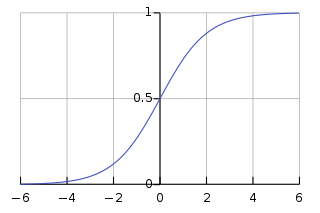Sigmoid function
• Equation: f(x) = s= 1/(1+e⁻ˣ)
• Derivative: f’(x) = s*(1-s)
• Range: (0,1)

Advantages:

1. The function is differentiable.That means, we can find the slope of the sigmoid curve at any two points.
2. Output values bound between 0 and 1, normalizing the output of each neuron.

Disadvantages:

1. Vanishing gradient — for very high or very low values of X, there is almost no change to the prediction, causing a vanishing gradient problem.
2. Due to vanishing gradient problem, sigmoids have slow convergence.
3. Outputs not zero centered.
4. Computationally expensive.

#### 2. Tan-h / Hyperbolic tangent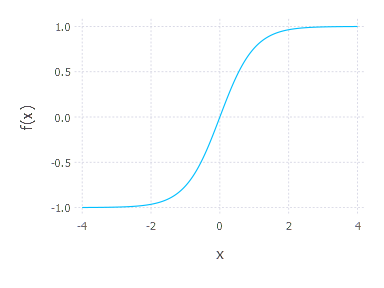Tan-h function
• Equation : f(x) = a =tanh(x) =(eˣ - e⁻ˣ)/(eˣ +e⁻ˣ)
• Derivative: (1- a²)
• Range: (-1, 1)

Advantages:

1. Zero centered — making it easier to model inputs that have strongly negative, neutral, and strongly positive values.
2. The function and its derivative both are monotonic.
3. Works better than sigmoid function

Disadvantage:

1. It also suffers vanishing gradient problem and hence slow convergence.

#### 3. ReLU (Rectified Linear Unit)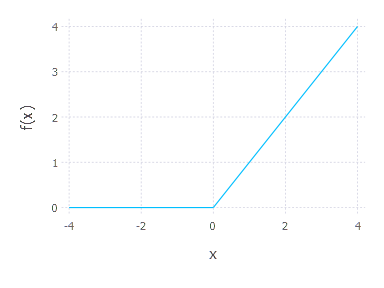ReLU function
• Equation: f(x) = a =max(0,x)
• Derivative: f’(x) = { 1 ; if z>0, 0; if z<0 and undefined if z=0 }
• Range: (0, +∞)

Advantages:

1. Computationally efficient — allows the network to converge very quickly
2. Non-linear — although it looks like a linear function, ReLU has a derivative function and allows for back-propagation

Disadvantages:

1. The Dying ReLU problem — when inputs approach zero, or are negative, the gradient of the function becomes zero, the network cannot perform back-propagation and cannot learn.

#### 4. Leaky ReLU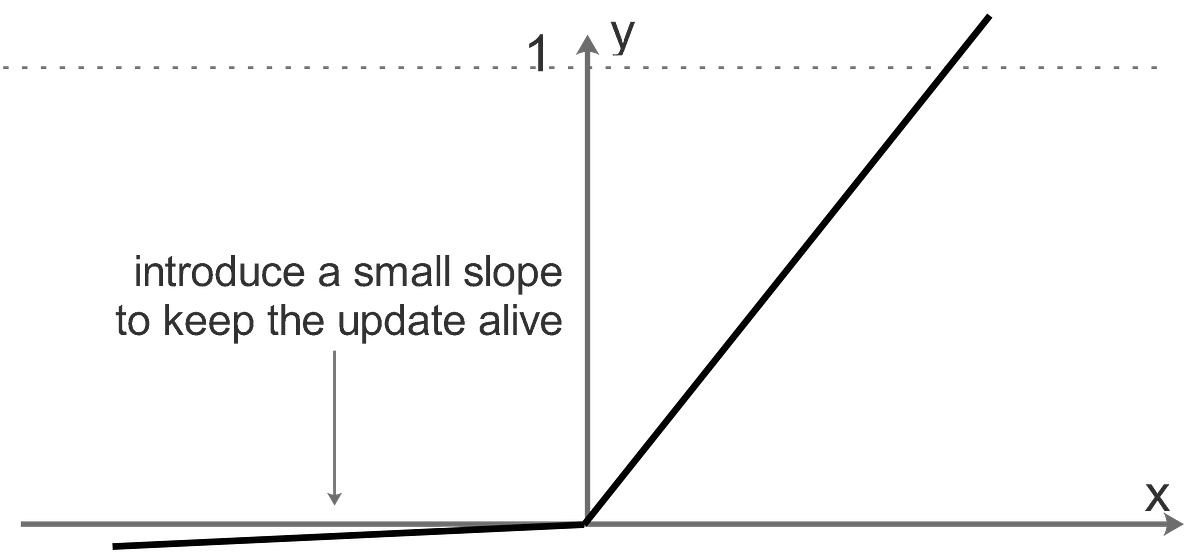Leaky ReLU
• Equation: f(x)= a = max(0.01x, x)
• Derivative: f’(x) = {0.01 ; if z<0, 1 ; otherwise}
• Range: (0.01, +∞)

Advantage:

1. Prevents dying ReLU problem — this variation of ReLU has a small positive slope in the negative area, so it does enable back-propagation, even for negative input values

Disadvantage:

1. Results not consistent — leaky ReLU does not provide consistent predictions for negative input values.
2. During the front propagation if the learning rate is set very high it will overshoot killing the neuron.

The idea of leaky ReLU can be extended even further. Instead of multiplying x with a constant term we can multiply it with a hyper-parameter which seems to work better the leaky ReLU. This extension to leaky ReLU is known as Parametric ReLU.

#### 5. Softmax

Softmax function calculates the probabilities distribution of the event over ’n’ different events.

• Equation: f(x) = eˣᵢ / (Σⱼ₌₀ eˣᵢ)
• Probabilistic interpretation: Sⱼ = P(y=j|x)
• Range: (0, 1)

Advantages:

1. Able to handle multiple classes only one class in other activation functions — normalizes the outputs for each class between 0 and 1, and divides by their sum, giving the probability of the input value being in a specific class.
2. Useful for output neurons — typically Softmax is used only for the output layer, for neural networks that need to classify inputs into multiple categories.

### 6. Which activation function to use?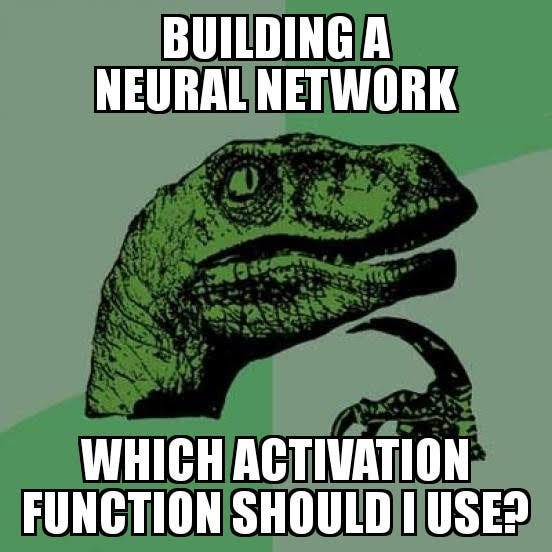Meme on Activation function

So till now we have seen different types of activation functions, their advantages and disadvantages. Now the question arises — Which activation function should I use for my neural network?

It would be incredibly difficult to recommend an activation function that works for all use cases. There are many considerations — how difficult it is to compute the derivative (if it is differentiable at all!), how quickly a network with your chosen AF converges, how smooth it is, whether it satisfies the conditions of the universal approximation theorem, whether it preserves normalization, and so on. You may or may not care about some or any of those.

• Sigmoid functions and their combinations generally work better in the case of classification problems.
• Sigmoid and tanh functions are sometimes avoided due to the vanishing gradient problem.
• Tanh is avoided most of the time due to dead neuron problem.
• ReLU activation function is widely used and is default choice as it yields better results.
• If we encounter a case of dead neurons in our networks the leaky ReLU function is the best choice.
• ReLU function should only be used in the hidden layers.
• An output layer can be linear activation function in case of regression problems.

Hope this article serves the purpose of getting idea about the activation function , why when and which to use it for a given problem statement. Comment down your views about the article and also click on Claps to encourage me for writing new articles.